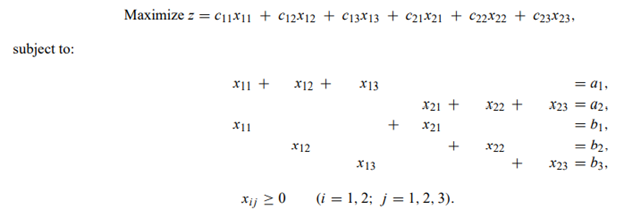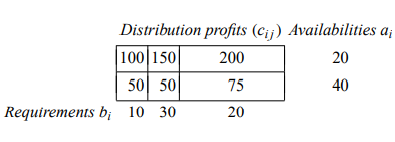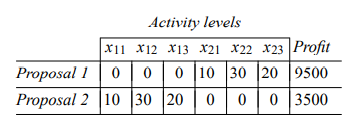# Consider a transportation problem for profit maximization

Consider a transportation problem for profit maximization:a) Suppose that we solve this problem by decomposition, letting the requirement bi constraints and nonnegativity xij ≥ 0 constraints compose the subproblem. Is it easy to solve the subproblem at each iteration? Does the restricted master problem inherit the network structure of the problem, or is the network structure ‘‘lost’’ at the master-problem level?

b) Use the decomposition procedure to solve for the data specified in the following tableInitiate the algorithm with two proposals:To simplify calculations, you may wish to use the fact that a transportation problem contains a redundant equation and remove the second supply equation from the problem.